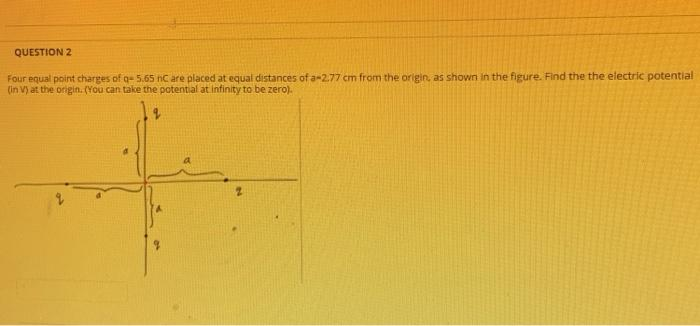### Create an Account

Home / Questions / Four equal point charges of q-5.65 n are placed at equal distances of a 2.77 cm from the ...

# Four equal point charges of q-5.65 n are placed at equal distances of a 2.77 cm from the origin, as shown in the figure. Find the the electric potential Un V) at the ongin.

Four equal point charges of q-5.65 n are placed at equal distances of a 2.77 cm from the origin, as shown in the figure. Find the the electric potential Un V) at the ongin.May 29 2021 View more View LessSubscribe To Get Solution